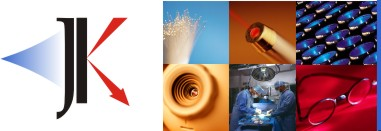Thin Film Design & Applications2 Layer AR Coatings (V-coat)

Benefits:  More material choices for index.  More refinement options.  Easy to
manufacture.

Limitations:  Narrow band.

Choosing Layer indices: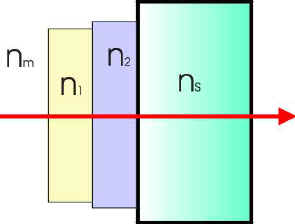A straight forward way of determining if the materials you have on hand, combined, would make a decent AR, is to use a Schuster diagram. (reference 1)

Below, the Schuster diagram has been calculated
for glass (n=1.52) & germanium (n=4.0) in an air medium (nm=1.0).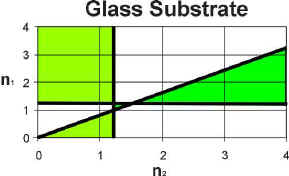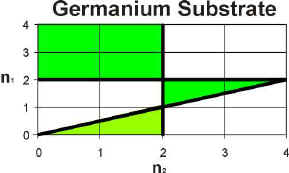The calculation requires that of the following three expressions: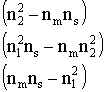All three must be positive
or,
Any two are negative and the third positive.

Layer thicknesses that are equal optical thickness (reference 2)

To calculate the refractive index requirments
for a 2 layer AR coating where both layers
are of equal optical thickness (each are QWOT's,
see terminology)
then the use the following: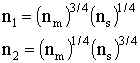Web www.kruschwitz.com